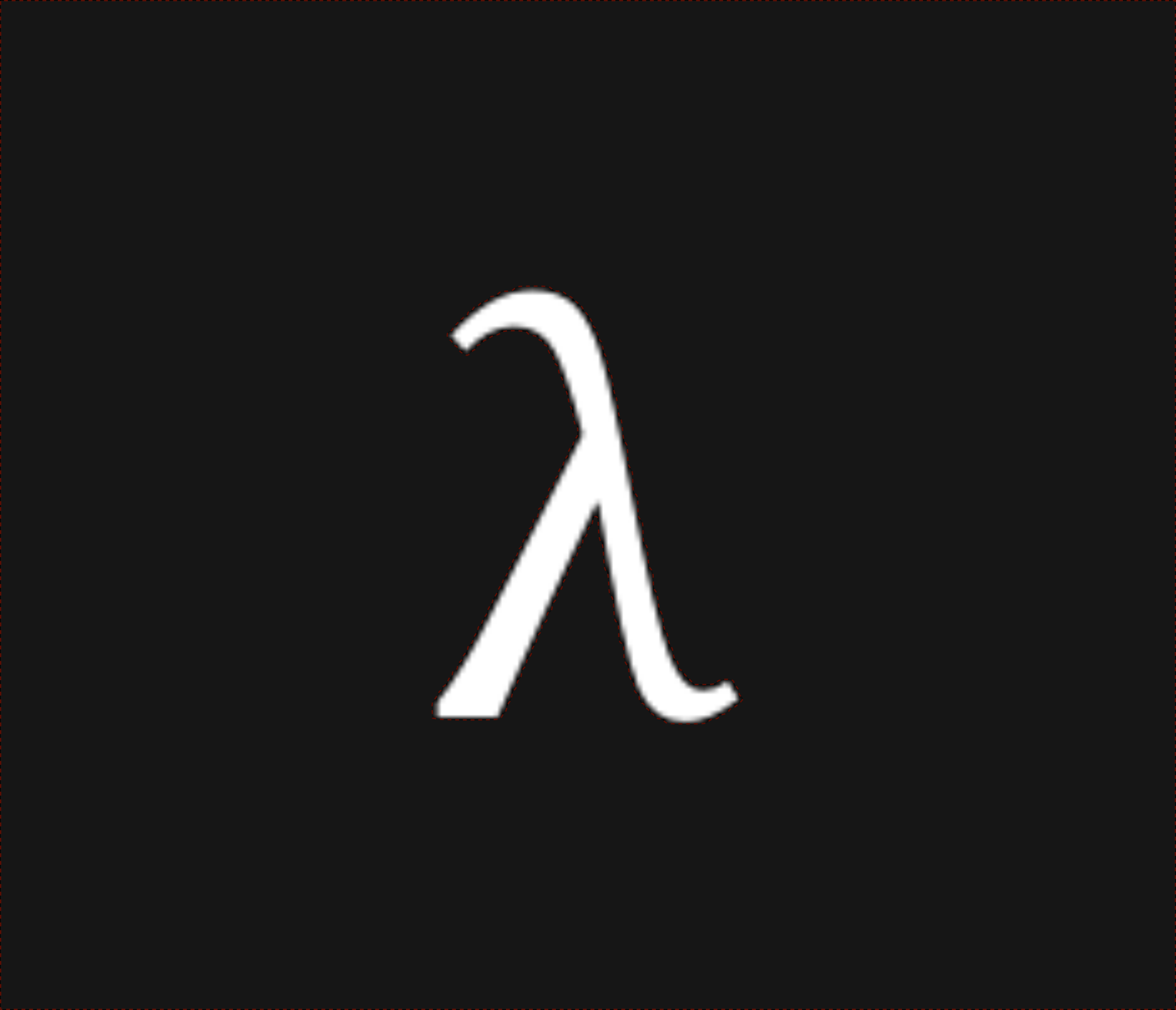nixpulvis

## Achilles' Tent Notes #11 written by Nathan Lilienthal on November 11, 2019.

### Parties & Protocols

• enum Other { Ip(...), Mem(...), ... }
• trait Protocol
• enum Party { Me, Other(Other), Protocol(Box<Protocol>) }

All of Me, Other and Protocol implement trait Party (maybe Entity).

#### Same source protocol, with CLI flags:

• A runs with --parties=[10.0.1.1, @me, 10.0.1.2]
• B runs with --parties=[@me, 10.0.1.0, 10.0.1.2]
• C runs with --parties=[10.0.1.1, 10.0.1.0, @me]
use achillies::party::Party;

// Could be a const-fn to allow compiling with specific
// parties established.
let [A, B, C]: [Party; 3] = parse_flags();
let P = init_protocol! {
GC(3);

Garbler   => [A, B],
Evaluator => C,
};

// Symmetric inputs
let [x,y,z]: [Obliv<u32>; 3] = P.conceals(i, P.parties);

// Asymmetric inputs
let [y,z] = P.conceals(gen_rand(), [B,C]);

Conceals Implementation, and Conceal Macros
// Lazy/party specific block?
let x = conceal!(A@{read_env()} => P);
let [y,z] = conceals!([B,C]@{gen_rand()} => P);

// NOTE: conceals may be implemented as:
fn conceals(self, v: T, parties: Vec<Party>) -> Vec<Obliv<T>> {
let xs = Vec::with_capacity(parties.len());
for party in parties.iter() {
xs.push(self.conceal(v, party));
}
xs
}

// Compute on Obliv data.
let r = f(x, y, z);

// Explicit reveals.
P.reveal(r, A);

Different source (C's shown)
use achillies::party::{Party, Other};

let P = init_protocol! {
GC(3);

Garbler => [
Party::Other(Other::Ip("10.0.1.1")),
Party::Other(Other::Ip("10.0.1.0")),
],
Evaluator => Party::Me,
};

// We don't have to know what the source of x and y is.
let x = P.conceal(None, A);
let y = P.conceal(None, B);
let z = P.conceal(Some(gen_rand()), C);

// Compute on Obliv data.
let r = f(x, y, z);

// Explicit reveals.
P.reveal(r, A);


### Merge Sort

We see that merge_sort over a slice of Obliv<T> is mostly recursive calls to a merge function, as we expect.

fn merge_sort<T>(slice: &[Obliv<T>]) -> Vec<Obliv<T>>
where T: PartialOrd + Clone
{
if slice.len() == 1 { return slice.to_owned() }
let mid   = slice.len() / 2;
let left  = merge_sort(&slice[0..mid]);
let right = merge_sort(&slice[mid..slice.len()]);
merge(&left, &right)
}


The merge function requires a bit of care to avoid leaking the original orders of it’s arguments.

// You start with an slice of Obliv<T> meaning you know
// essentially nothing but the length of the slice. After merging,
// you end up with a Vec of Obliv<T> again meaning that you
// know nothing but the length. This means this function **should
// not** leak, for instance, the order of the elements in the
// original slice. See leaky_merge.
fn merge<T>(left: &[Obliv<T>], right: &[Obliv<T>]) -> Vec<Obliv<T>>
where T: PartialOrd + Clone
{
let out_len = left.len() + right.len();
let mut out = Vec::with_capacity(out_len);

// Both li and ri must be Obliv types, because
// they will need to be mutated inside the obliv if, which
// is disallowed otherwise.  This makes sense, because
// inspection of the current left and right slice index while
// running the following for loop would leak the ....
//
// The Obliv type is public information at first however,
// since it's well established that any instance of this
// sorting algorithm starts at the 0th index of each slice.
let mut li = Obliv::public(0);
let mut ri = Obliv::public(0);

// We need to use the length's of the slices in our obliv if
// logic, so we must make an explicit public left_len obliv
// value.
let left_len = Obliv::public(left.len());
let right_len = Obliv::public(right.len());

for i in 0..out_len {
out.push({
obliv if li == left_len ||
ri < right_len &&
Oram(right)[ri] > Oram(left)[li]
{
let o = Oram(right)[ri].clone();
ri += Obliv::public(1);
o
} else {
let o = Oram(left)[li].clone();
li += Obliv::public(1);
o
}
});
}
out
}

Leaky merge function

We show a simple mistake, and where our type system would catch it.

fn leaky_merge<T: PartialOrd>(left: &[Obliv<T>], right: &[Obliv<T>])
-> Vec<Obliv<T>>
{
let out_len = left.len() + right.len();
let mut out = Vec::with_capacity(out_len);

// Mistake here, we would leak the original order by allowing
// inspection of the left and right index we use throughout
// the algorithm.
let mut li = 0;
let mut ri = 0;

for i in 0..out_len {
let r = right[ri].clone();
let l = left[li].clone();

out.push({
// NOTE: This luckily type-fails here. We can't mutate
// a plain value within an obliv if.
//
// We *could* use an always block to allow mutation.
// However, then the index would *always* increment by
// 1, breaking the merge algorithm.
obliv if li == left.len() ||
ri < right.len() &&
right[ri] > left[li]
{
let o = right[ri].clone();
ri += 1;
o
} else {
let o = left[li].clone();
li += 1;
o
}
});

// We didn't declare obliv index (aka linear scan), so
// this leaks the original order.
println!("{}", li, ri);
}
out
}


### TODO

• GC(3) can be anyParty::Protocol trait or something…?
• Exact conceal and conceals semantics in multi-party setting.
• Short circuit semantics for ||
• Example  fn median<T: PartialOrd>(inputs: Vec<Vec<Obliv<T>>>) -> T
• Example  fn stable_match